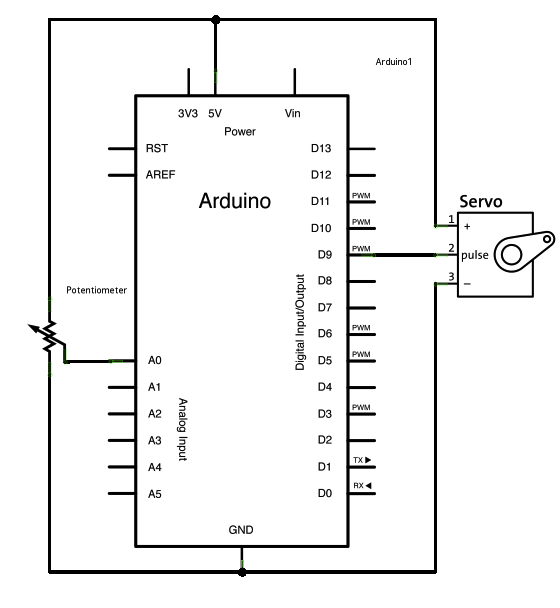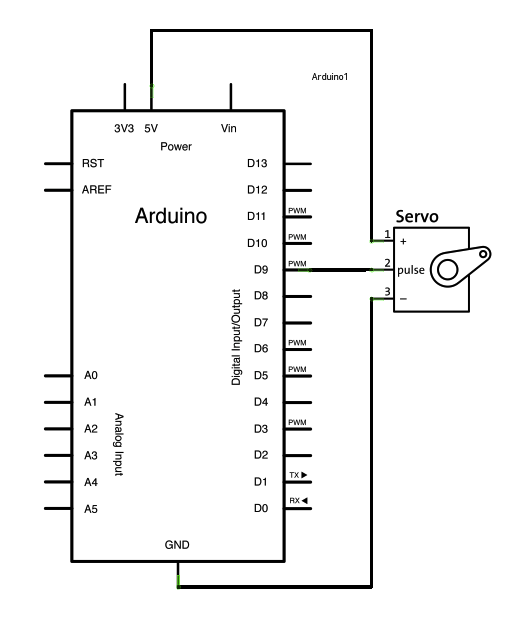# Arduino Servo Motor Controlling

Author : Dinesh Kumar Wickramasinghe

Introduction and Images

Hello friends, This tutorial is about controlling servo motors with your favorite Microrontroller board Arduino. Servo motor is an essential part of all robotics lovers for requirements like robotics arms controlling. And also you do not need any intermediate controller IC s when you are dealing with Arduino. That means you can directly connect your servo motor to Arduino.

Here are two images of my setup.

Since there are several websites on Internet which explains the functionality of the Servo motor, I am not going to explain it here (Please see the links provided in the end of this article). Giving you only the source code samples. This code is based on the Arduino official tutorial of Servo Motor controlling. You can also find these examples from the examples code library of Arduino IDE.

There are basically two examples called "Knob" and "Sweep"

The "Knob" example is to control the angle of the Servo by using a Potentio meter. Once you read the Potentio meter value using the analogRead() function, then you will get minimum of 0 and Maximum of 1023 values. But to set the servo angle, we need to pass a value between 0 and 180. So, to map the ranges, the code uses the map() function. Here is the schematic and the sketch (source code).```/*
Controlling a servo position using a potentiometer (variable resistor)
by Michal Rinott

modified on 8 Nov 2013
by Scott Fitzgerald
http://www.arduino.cc/en/Tutorial/Knob
*/

#include <Servo.h>

Servo myservo;  // create servo object to control a servo

int potpin = 0;  // analog pin used to connect the potentiometer
int val;    // variable to read the value from the analog pin

void setup() {
myservo.attach(9);  // attaches the servo on pin 9 to the servo object
}

void loop() {
val = analogRead(potpin);            // reads the value of the potentiometer (value between 0 and 1023)
val = map(val, 0, 1023, 0, 180);     // scale it to use it with the servo (value between 0 and 180)
myservo.write(val);                  // sets the servo position according to the scaled value
delay(15);                           // waits for the servo to get there
}
```

Other example is "Sweep". This example code will continuasly rotate the servo from 0 to 180 degrees and backwords. Below is the schematic and the source code for "Sweep".```/* Sweep
by BARRAGAN
This example code is in the public domain.

modified 8 Nov 2013
by Scott Fitzgerald
http://www.arduino.cc/en/Tutorial/Sweep
*/

#include <Servo.h>

Servo myservo;  // create servo object to control a servo
// twelve servo objects can be created on most boards

int pos = 0;    // variable to store the servo position

void setup() {
myservo.attach(9);  // attaches the servo on pin 9 to the servo object
}

void loop() {
for (pos = 0; pos <= 180; pos += 1) { // goes from 0 degrees to 180 degrees
// in steps of 1 degree
myservo.write(pos);              // tell servo to go to position in variable 'pos'
delay(15);                       // waits 15ms for the servo to reach the position
}
for (pos = 180; pos >= 0; pos -= 1) { // goes from 180 degrees to 0 degrees
myservo.write(pos);              // tell servo to go to position in variable 'pos'
delay(15);                       // waits 15ms for the servo to reach the position
}
}
```